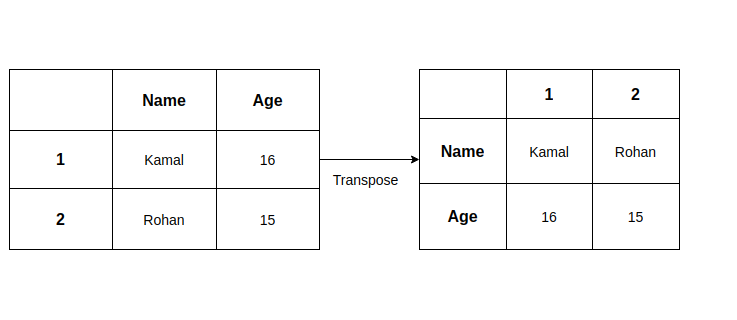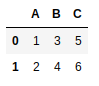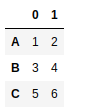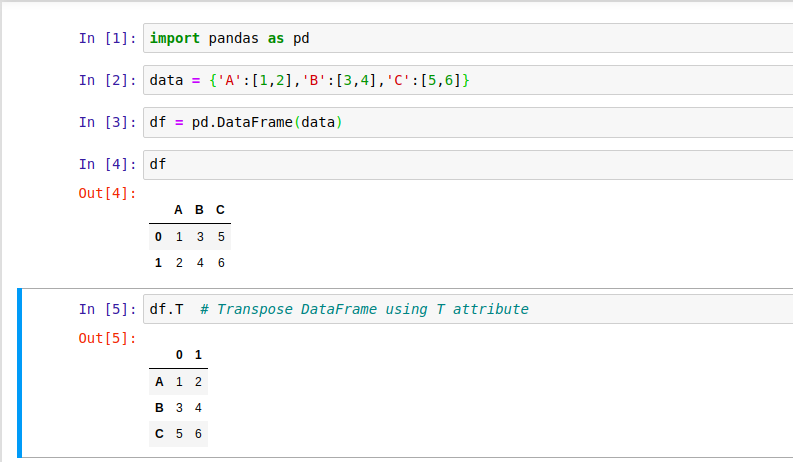# Pandas DataFrame: pandas DataFrame transpose() Method

To transpose a DataFrame, Pandas provides transpose() method and a T attribute both belong to DataFrame. Transpose of a DataFrame (which is somehow 2D array) is a process of changing rows as columns and columns as rows. For example, you can understand it by the below image of a DataFrame that contains name and age of students in row-column format (tabular format).

In the first image, name and age are columns while in the second image both columns are transposed to rows.## How to Transpose a DataFrame?

Pandas DataFrame provides mainly two ways to transport a DataFrame. These two ways are:

1. DataFrame.T Attribute
2. DataFrame.transpose() Method

## Pandas DataFrame.T Attribute

It is a property (attribute) of DataFrame that transposes index (rows) and columns. It is an accessor to the transpose() method of DataFrame. The syntax of property T is given below.

``DataFrame.T``

## Example: Transpose DataFrame by using T attribute

Here, we are creating a DataFrame from a dictionary and after that transposing it by using the T attribute of DataFrame.

``````import pandas as pd
# Take a dictionary
data = {'A':[1,2],'B':[3,4],'C':[5,6]}
# Create a DataFrame
df = pd.DataFrame(data)
print(df)
# Transpose the DataFrame
print("Data After Transposing...")
df_t = df.T
print(df_t)``````

Output:Data After Transposing...## Jupyter Notebook

If you are familiar with Jupyter and using Jupyter notebook to practice the Pandas then the above example can be executed like below.

If you are a beginner and not aware of Jupyter notebook then we recommend you skip this step and scroll down to read further.## Pandas DataFrame.transpose() Method Syntax

``DataFrame.transpose(self, *args, copy: bool = False)``

It is another way to transpose a DataFrame. The transpose() method returns a DataFrame by replacing row as columns and vice-versa. It contains several parameters that are given below.

## DataFrame.transpose() Method Parameters:

 Name Description *args tuple, optional Accepted for compatibility with NumPy. copy It is bool type and the default value is False. It is used to specify whether to copy the data after transposing. It is always required for mixed dtype DataFrames, or for DataFrames with any extension types.

## Transposing DataFrame by using transpose() Method

Here, we are creating a DataFrame by using a dictionary and after that transposing it by using the transpose() method.

``````import pandas as pd
# Take a dictionary
data = {'A':[1,2],'B':[3,4],'C':[5,6]}
# Create a DataFrame
df = pd.DataFrame(data)
print(df)
# Transpose the DataFrame
print("Data After Transposing...")
df_t = df.transpose()
print(df_t)``````

Output:Data After Transposing...Note: By default value of the copy parameter is False.

## Example: Transpose DataFrame

Here, we are not setting a copy parameter for the transpose() method. By default, the copy is set to False which means DataFrame created after transpose, points to the original DataFrame's values. It means if we make any change to original DataFrame, changes reflect newly transposed DataFrame too.

``````import pandas as pd
# Take a Dictionary
data = {'A':[1,2],'B':[3,4],'C':[5,6]}
# Create a DataFrame
df = pd.DataFrame(data)
print(df)
# Transpose the DataFrame
df_Trans = df.transpose()
print("After Transposing...")
print(df_Trans)
# Modifying the Dataframe
df.iat[0,0] = 10
print("After Modifying...")
print(df)
print("Transposed DataFrame")
print(df_Trans) # Transposed DataFrame got modified``````

Output:

A  B  C
0  1  3  5
1  2  4  6
After Transposing...
0  1
A  1  2
B  3  4
C  5  6
After Modifying...
A  B  C
0  10  3  5
1   2  4  6
Transposed DataFrame
0  1
A  10  2
B   3  4
C   5  6

Note: In the above example, we modified the value of the original DataFrame only but it reflects the transposed DataFrame as well.

## Example: Transpose DataFrame by Setting copy=True

If we set copy parameter to True then Pandas create a separate new copy of DataFrame as a transpose result. It gives one advantage that if we change any value of original DataFrame then the transposed DataFrame does not affect.

``````import pandas as pd
# Take a Dictionary
data = {'A':[1,2],'B':[3,4],'C':[5,6]}
# Create a DataFrame
df = pd.DataFrame(data)
print(df)
# Transpose the DataFrame
df_Trans = df.transpose(copy=True)
print("After Transposing...")
print(df_Trans)
# Modifying the Dataframe
df.iat[0,0] = 10
print("After Modifying...")
print(df)
print("Transposed DataFrame")
print(df_Trans) # Transposed DataFrame got modified``````

Output:

A  B  C
0  1  3  5
1  2  4  6
After Transposing...
0  1
A  1  2
B  3  4
C  5  6
After Modifying...
A  B  C
0  10  3  5
1   2  4  6
Transposed DataFrame
0  1
A  1  2
B   3  4
C   5  6

### Conclusion

Well, in this topic, we have learned to transpose a DataFrame with the help of several examples. We Used transpose() method and T attribute of DataFrame that are used to get transposed DataFrame.

If we missed something, you can suggest us at - [email protected]

Trending